# MSBSHSE Class 8 Science Chapter 5 Inside the Atom Textbook Solutions

In MSBSHSE Class 8 Science Chapter 5 Inside the Atom, students learn about what comprises inside an atom. Here, we present you the important questions and solutions of the chapter that will help students to get an in-depth understanding of all the essential concepts and master it confidently.

All the chapter-wise explanations of important questions and solutions of MSBSHSE Class 8 Science have been covered by our subject matter experts. We hope that students can benefit from it and learn new concepts, while they’re gearing up for the MSBSHSE Class 8 2020 exam. All the important questions of the MSBSHSE Class 8 Science Solutions for Chapter 5 Inside the Atom have been thoroughly covered to help students to grasp a rigorous knowledge of important concepts in a lucid manner.

In this article, we have extensively covered all the exercise questions and added a few additional questions, along with solutions for Chapter 5 from the MSBSHSE Class 8 Science Syllabus. Class 8 students can check the questions while studying this chapter and practice from it to build a rock-solid foundation of key concepts that are discussed and score good marks in this subject.

## Maharashtra Board Class 8 Science Chapter 5: BYJU’S Important Questions & Answers

### MSBSHSE Class 8 Science Chapter 5 Textbook Exercise Questions

a. What is the difference in the atomic models of Thomson and Rutherford?

b. What is meant by valency of an element? What is the relationship between the number of valence electrons and valency?

c. What is meant by atomic mass number? Explain how the atomic number and mass number of carbon are 6 and 12, respectively.

d. What is meant by subatomic particle? Give brief information of three subatomic particles with reference to electrical charge, mass and location.

Answer: a. Differences between Thomson and Rutherford Theory are listed below:

 Thomson Theory Rutherford Theory 1. This theory states that the negatively charged electron are entrenched in a positively charged solid material which has a spherical shape This theory states that an atom consists of a positively charged nucleus around which the electrons revolve in an orbit 2. It does not divulge any details about the atomic nucleus It mentions about the atomic nucleus 3. In this theory, it states that electrons are distributed in an atom in a uniform manner It states that electrons revolve around a solid material at the centre i.e. atomic nucleus 4. This theory indicates that an atom is spherical in shape This theory indicates that an atom has a solid core at the centre defined as the nucleus which is surrounded by the electrons 5. Nowhere in this theory does it mention the constituents of nucleus This theory clearly mentions that nucleus is a composition of neutrons and protons

b. Valency of an element: It refers to the number of electrons of an atom of the element that is used to combine or mix with the atoms of other elements. It is the combining capacity of an atom of the respective element.

Here’s the relationship between the number of valence electrons and valency:

Valency of an element can be determined by its electronic configuration. It explains about the gain or loss in the total number of electrons in order to derive at the closest noble gas configuration.

For example:

The electronic configuration of Sodium (Na) is 11 i.e. 2, 8, 1. Hence, the valency of Na is 1.

The electronic configuration of Chlorine (Cl) is 17 i.e. 2, 8, 7. Hence, its valency is 1.

Number of valence electrons: The outermost shell of an atom contains the total number of electrons in it. However, it is not mandatory that all the valence electrons would be involved in bonding.

For example:

The electronic configuration of Sodium (Na) is 11 i.e. 2, 8, 1. Hence, the number of valence electrons is 1.

The electronic configuration of Chlorine (Cl) is 17 i.e. 2, 8, 7. Hence, its number of valence electrons is 7.

Hence, we have observed that valency of an element is directly proportional to the number of valence electrons in that atom.

c. Atomic Mass Number: It is the sum of the total number of neutrons and protons present in an atomic nucleus.

Atomic mass number = Number of neutrons + Number of protons

Atomic number is denoted by Z = Number of protons = Number of electrons.

Mass number is denoted by A = Number of protons + Number of neutrons.

For example : In a carbon atom the total number of protons = 6

Number of neutrons = 6

Number of electron = 6

Atomic number (Z) = number of protons = number of electrons = 6.

Mass number (A) = number of protons + number of neutrons = 6 + 6 = 12.

d. Subatomic particles: A subatomic particle is a functional and structural unit of matters. This implies that all the matters are a combination of these fundamental particles. As per the modern atomic theory, an atom has a nucleus at its center or core. This nucleus contains subatomic particles such as protons and neutrons in it.

Difference between subatomic particles are listed below:

Electron

1. Electrons are usually present outside the atom nucleus.

2. They are negatively charged i.e (1.6 × 10-19 Coulomb).

3. The mass of an electron is considered as negligible as it’s 1800 times less than that of hydrogen.

4. Relative mass = 1/ 1840 times hydrogen

5. It revolves around the nucleus of an atom in a discrete orbit.

Proton

1. Protons are present inside the nucleus of an atom.

2. Protons are positively charged i.e. (1.6 × 10-19 Coulomb).

3. The mass of a proton is approximately 1u (1Dalton) i.e. (1u = 1.66 × 10-27 g).

4. They are bound in the nucleus closely.

Neutron

1. Neutrons are present in an atom nucleus.

2. Neutrons have no charge, hence they are neutral.

3. The mass of a neutron is nearly equal to the mass of a proton that is 1u (1Dalton) i.e. (1u = 1.66 × 10-27 g).

4. They are bound in the nucleus closely.

Question 2: Give scientific reasons:

a. All the mass of an atom is concentrated in the nucleus.

b. Atom is electrically neutral.

c. Atomic mass number is a whole number.

d. Atoms are stable though negatively charged electrons are revolving within it.

Answer: a. All the mass of an atom is concentrated in the nucleus because an atom consists of three subatomic particles such as electron, proton and neutron. The nucleus present at the centre of an atom usually contains two subatomic particles i.e. protons and neutrons. The total mass of a nucleus is the sum of the mass of protons and neutrons located at the central part of an atom.

b. An atom is electrically neutral because there are electrons and protons in an atom which carry charges and each atom has an equal number of protons (positively charged) and electrons (negatively charged) particles.

c. An atomic mass number is a whole number because it is the cumulative sum of the total number of protons and neutrons present in an atom, which is represented in the form of integers.

d. Atoms are stable irrespective of the fact that negatively charged electrons are revolving within it because each atom consists of equal numbers of protons and electrons. Hence, the charge on negatively charged electrons are balanced by charge on the positively charged proton. Therefore, an atom is electrically neutral and stable at all times.

Question 3: Define the following forms

a. Atom

b. Isotope

c. Atomic number

d. Atomic mass number

e. Moderator in nuclear reactor

Answer: a. Atom: An atom is defined as the structural and functional unit of matter. It is the smallest particle of an element which retains its chemical identity in all the physical and chemical changes. The term “atom” comes from the Greek word ‘Atomos’, which means that which cannot be divided because atoms are the smallest and minutest things in the universe which cannot be divided. Atoms comprise three subatomic particles like protons, neutrons and electrons.

b. Isotopes: Isotopes are the atoms that have the same atomic number but different mass number. As a matter of fact, isotopes have the same number of protons but different numbers of neutrons in it.

Some common examples of isotopes include carbon has three isotopes, namely, C – 12, C – 13, C – 14. The mass number of isotopes is also represented by another method as 12C , 13C and 14C. The isotopes have the same proton number but different neutron number.

c. Atomic number: Atomic number is equal to the total numbers of electrons in an atom. The number of protons in the nucleus of an atom are characteristic of a chemical element and determine its place in the periodic table.

For example :

Atomic number of carbon = 6

Atomic number of oxygen = 8

d. Atomic Mass Number: It is the sum of the total number of protons and neutrons present in an atomic nucleus.

Atomic mass number = number of protons + number of neutrons.

Atomic number is denoted by Z = number of protons = number of electrons.

Mass number is denoted by A = number of protons + number of neutrons.

For example : In a carbon atom, Number of protons = 6

Number of neutrons = 6

Number of electrons = 6

Atomic number (Z) = number of protons = number of electrons = 6

Mass number (A) = number of proton + number of neutrons = 6 + 6 =12

e. Moderator in nuclear reactor: Moderator of a nuclear reactor is a substance that slows or brings down the neutrons speed. In traditional and ancient nuclear reactors, the moderator is considered as similar to that of coolant like water.

Question 4: Draw a neat labelled diagram

a. Rutherford’s scattering experiment

b. Thomson’s atomic model

c. Diagramatic sketch of electronic configurations of magnesium (Atomic number 12)

d. Diagramatic sketch of electronic configuration of Argon (Atomic number 18)

a. Rutherford’s scattering experiment: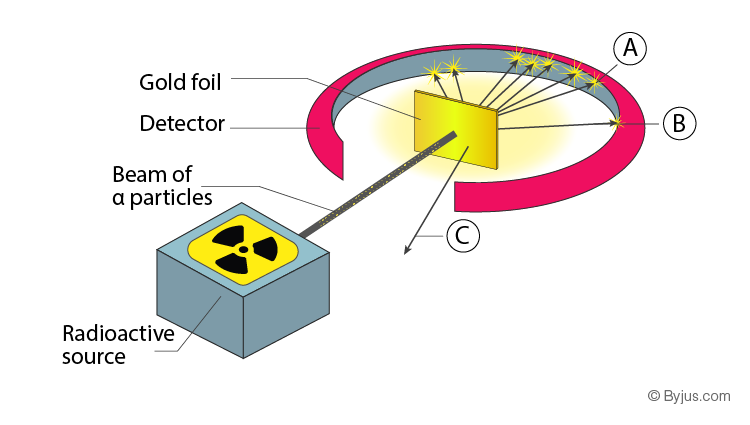b. Thomson’s atomic model: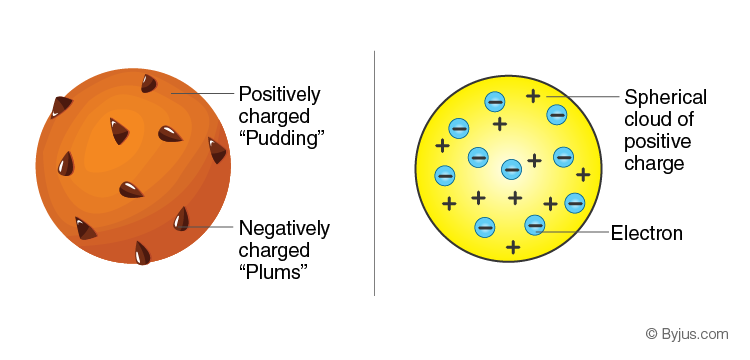c. Diagramatic sketch of electronic configurations of Magnesium (Atomic number 12):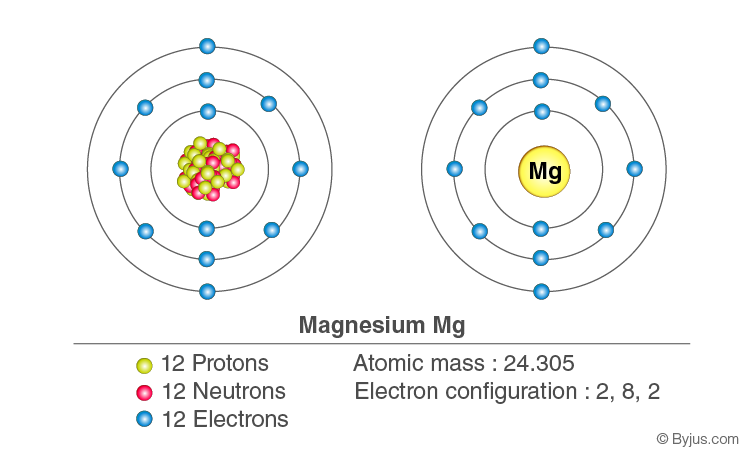d. Diagramatic sketch of electronic configuration of Argon (Atomic number 18):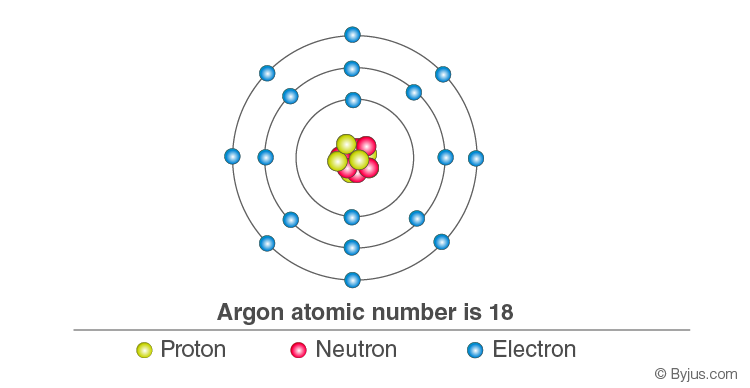Question 6: Fill in the blanks:

a. Electrons, protons, neutrons are the types of _________ in an atom.

b. An electron carries a _________charge.

c. The electron shell _____________ is nearest to the nucleus.

d. The electronic configuration Magnesium is 2, 8, 2. From this it is understood that the valence shell of Magnesium is_____________

e. The valency of hydrogen is ‘one as per the molecular formula H2O. Therefore, valency of ‘Fe’ turns out to be ______________ as per the formula Fe2O3.

a. Electrons, protons, neutrons are the types of subatomic particles in an atom.

b. An electron carries a negative charge.

c. The electron shell K is nearest to the nucleus.

d. The electronic configuration Magnesium is 2, 8, 2. From this it is understood that the valence shell of Magnesium is M shell.

e. The valency of hydrogen is ‘one as per the molecular formula H2O. Therefore, valency of ‘Fe’ turns out to be three as per the formula Fe2O3.

Question 6: Match the pairs:

 Group ‘A’ Group ‘B’ a. Proton 1. Negatively charged b. Electron 2. Neutral c. Neutron 3. Positively charged

 Group ‘A’ Group ‘B’ a. Proton 1. Positively charged b. Electron 2. Negatively charged c. Neutron 3. Neutral

Question 7: Deduce from the datum provided.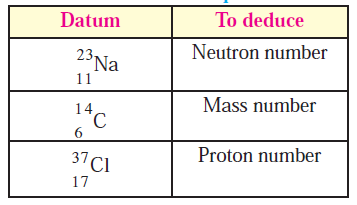(i) Neutron number = Mass number – Number of protons

= 23 – 11

= 12

(ii) Mass number = 14

(iii) Proton number = Atomic number

= 17

### MSBSHSE Class 8 Science Chapter 5 Additional Questions

Question 1: What is meant by matter?

Answer: Matter is everything that is around us, such as atoms and compounds are all composed of the smallest parts of matter. Matter is made of molecules which in turn, are formed from atoms.

Question 2: Describe matter as stated by the great Indian philosopher Acharya Kanad.

Answer: The great Indian sage and philosopher, Acharya Kanad (6th century B.C.) stated that there is a limit to divide matter into small particles. He named the indivisible particles that constitute matter as ‘Paramanu’ which means the smallest particles. He also stated that ‘Paramanu’ is indestructible by nature.

Question 3: Who coined the term ‘Atom’?

Answer: The Greek philosopher Democritus who lived in the 5th century B.C. stated that matter is made of very small particles which cannot be divided. Democritus described the smallest particle of matter and named it as ‘Atom’ or ‘Atomos’ which means the one which cannot be cut or divided.

Question 4: Describe Dalton’s Atomic Theory.

Answer: In 1803 A.D. John Dalton, the British scientist proposed ‘Atomic Theory’. According to this theory, matter is composed of atoms which are indivisible and indestructible. All atoms of an element are alike while different elements have different atoms with different mass.

Question 5: Describe Thomson’s plum pudding model of atom.

Answer: In 1904, J.J. Thomson demonstrated the first model of atomic structure through the plum pudding model of atom. According to this model, the positive charge is distributed throughout the atom while the negatively charged electrons are embedded in it. There is a balance between the distributed positive charge by the negative charge on the electrons. Due to this reason, the atom becomes electrically neutral.

Question 6: Describe Rutherford’s Nuclear atomic model.

Answer: Given below are the postulates of Rutherford’s Nuclear atomic model:

1. There is a positively charged nucleus at the centre of an atom.

2. The entire mass of the atom is almost concentrated in the nucleus.

3. The negatively charged particles are called electrons that revolve around the nucleus.

4. The total negative charge on all the electrons is equal to the positive charge on the nucleus. As the opposite charges are balanced the atom is electrically neutral.

5. There is an empty space between the revolving electron and the atomic nucleus.

Question 7: What is the shortcoming of Rutherford’s Nuclear atomic model?

Answer: One of the major shortcomings of Rutherford’s Nuclear atomic model is that it turns out to be unstable. However, in reality, all atoms except the radioactive atoms are stable.

Question 8: Describe Bohr’s stable orbit atomic model.

Answer: In 1913 Niels Bohr, a Danish scientist explained the stability of an atom by demonstrating the stable orbit atomic model. The important postulates of Bohr’s atomic model are listed as below:

(i) The electrons that revolve around the atomic nucleus lie in the concentric circular orbit at a certain distance from the nucleus.

(ii) The energy of an electron is constant while it is in a particular circular orbit.

(iii) When an electron jumps from an inner orbit to an outer orbit it absorbs energy equal to the difference of its energy level. Also, when it jumps from an outer orbit to an inner orbit it emits energy equal to the difference of its energy level.

Question 9: Describe nucleus.

Answer: The nucleus of an atom is positively charged. The entire mass of the atom is almost concentrated in the nucleus. The nucleus contains two types of subatomic particles together which are called nucleons. The two types of nucleons are protons and neutrons.

Question 10: Define Proton.

Answer: Proton is a positively charged subatomic particle in the atomic nucleus. The positive charge on the nucleus is due to the proton in it. A proton is represented by the symbol ‘p’. Each proton carries a positive charge of +1e. (1e = 1.6 × 10-19 coulomb).

Question 11: What is Neutron?

Answer: Neutron is an electrically neutral subatomic particle of an atom that is denoted by the symbol ‘n’. The number of neutrons in an atomic nucleus is denoted by the symbol ‘N’. Atomic nuclei of all the elements except hydrogen with atomic mass 1u, contain neutrons. The mass of a neutron is approximately 1u, which is almost equal to that of a proton.

Question 12: What comprises the extranuclear part?

Answer: The extranuclear part in an atomic structure basically includes the electrons that revolve around the nucleus and the empty space in between the nucleus and the electrons.

Question 13: What is an electron?

Answer: Electron is a negatively charged subatomic particle of an atom that is denoted by the symbol ‘e’. Each electron carries one unit of negative charge (-1e). Mass of an electron is 1800 times less than that of a hydrogen atom. Therefore, the mass of an electron can be treated as negligible.

Question 14: What is electronic configuration of an element?

Answer: According to their maximum capacity, the electrons in an atom are distributed in the shells. The shell wise distribution of the electron in an atom of an element is called the electronic configuration of that element. Each electron has a definite energy according to the shell in which it is present.

Question 15: Define valence electrons.

Answer: An atom forms chemical bonds from its outermost shell by using electrons. Valency of an atom is determined by the configuration of its outermost shell. Therefore, the outermost shell is known as the valence shell and the electrons in the outermost shell are called valence electrons.

Question 16: What are the uses of Isotopes?

Answer: Some elements have isotopes which are radioactive. They are used in a variety of fields such as agriculture, industry, medicine, research and development.

1. Uranium – 235 is particularly used for production of electricity and nuclear fission.

2. Radioactive isotopes like Cobalt – 60 are used in the medical treatment of life-threatening diseases such as cancer.

3. Iodine -131 is used to treat diseases such as goiter and thyroid gland disorders.

4. The radioactive isotopes are used for detection of cracks or leakage in the underground

Pipes such as Sodium -24.

5. Radioactive isotopes are used for preserving food from microbes.

6. The radioactive isotope C-14 is used for determining the age of archaeological objects.

Question 17: Give examples of moderators.

Answer: Heavy water or Graphite are used as moderators for reducing the speed of neutrons in an atom in a nuclear reactor.

Question 18: What is a nuclear reactor?

Answer: Nuclear reactor is a machine that is used to generate electricity on a large scale by using atomic energy. In a nuclear reactor, the nuclear energy in an atom is released by combining and bringing the nuclear reactions on the nuclear fuel.

Question 19: What is the function of a controller in a nuclear reactor?

Answer: A controller is used in a nuclear reactor to reduce the number of neutrons by absorbing the rods of boron, cadmium, beryllium, etc.

Question 20: How to control chain reaction in a nuclear reactor?

Answer: A chain reaction is usually kept under control to prevent a probable explosion. In order to control a chain reaction in the nuclear reactor it is necessary to slow down the speed and number of neutrons. For this purpose, moderators and controllers are used in a nuclear reactor.

We hope that the above mentioned solutions of “MSBSHSE Class 8 Science Chapter 5 Inside the Atom” will help students to build a strong and solid foundation of the different concepts mentioned in the chapter.

## Frequently Asked Questions on Maharashtra State Board Solutions for Class 8 Science Chapter 5 Inside the Atom

### How can I get Maharashtra State Board Class 8 Science Solutions Chapter 5 Inside the Atom?

Maharashtra State Board Class 8 Science Solutions Chapter 5  Inside the Atom is available as a scrollable PDF format online. We have also provided a clickable link for students to access the solution. Additionally, these questions and the solutions can be found on our webpage.

### Maharashtra State Board Class 8 Science Solutions Chapter 5: Why are they important?

Students are encouraged to solve these solutions as they are ideal guides for those preparing for the Class8 exams. These solutions provide answers to questions that are likely to be asked in the final exams. These solutions can assist students in scoring well in exams by helping them prepare. By identifying the errors early, the students can avoid making them during the exam.

### How to solve Maharashtra State Board Class 8 Science Solutions Chapter 5?

Students are encouraged to solve these questions first and then refer back to the solutions to get an idea about the answers. They can identify the mistakes and avoid them in the exams. Solving the solutions on time helps them to manage time better also to gauge their exam preparations.